# Frank Solutions Class 9 Maths Chapter 25 Surface Areas and Volume of Solids

Frank Solutions for Class 9 Maths Chapter 25 Surface Areas and Volume of Solids provide answers in an elaborate manner for exercise problems in the textbook. The solutions are prepared by the subject experts at BYJU’S after conducting vast research on each topic based on the latest syllabus of the ICSE Board. The main purpose of these solutions is to help students boost their exam preparation and score high in the final exam.

Chapter 25, Surface Areas and Volume of Solids, deals with the study of finding surface areas and volumes of combinations of solids. The answers are developed by subject experts to match Class 9 students’ understanding abilities. By practising these solutions, they can solve different types of questions that will appear in the annual exam easily. Students can download the solutions in PDF and practise offline as well from the link below.

## Frank Solutions for Class 9 Maths Chapter 25 Surface Areas and Volume of Solids Download PDF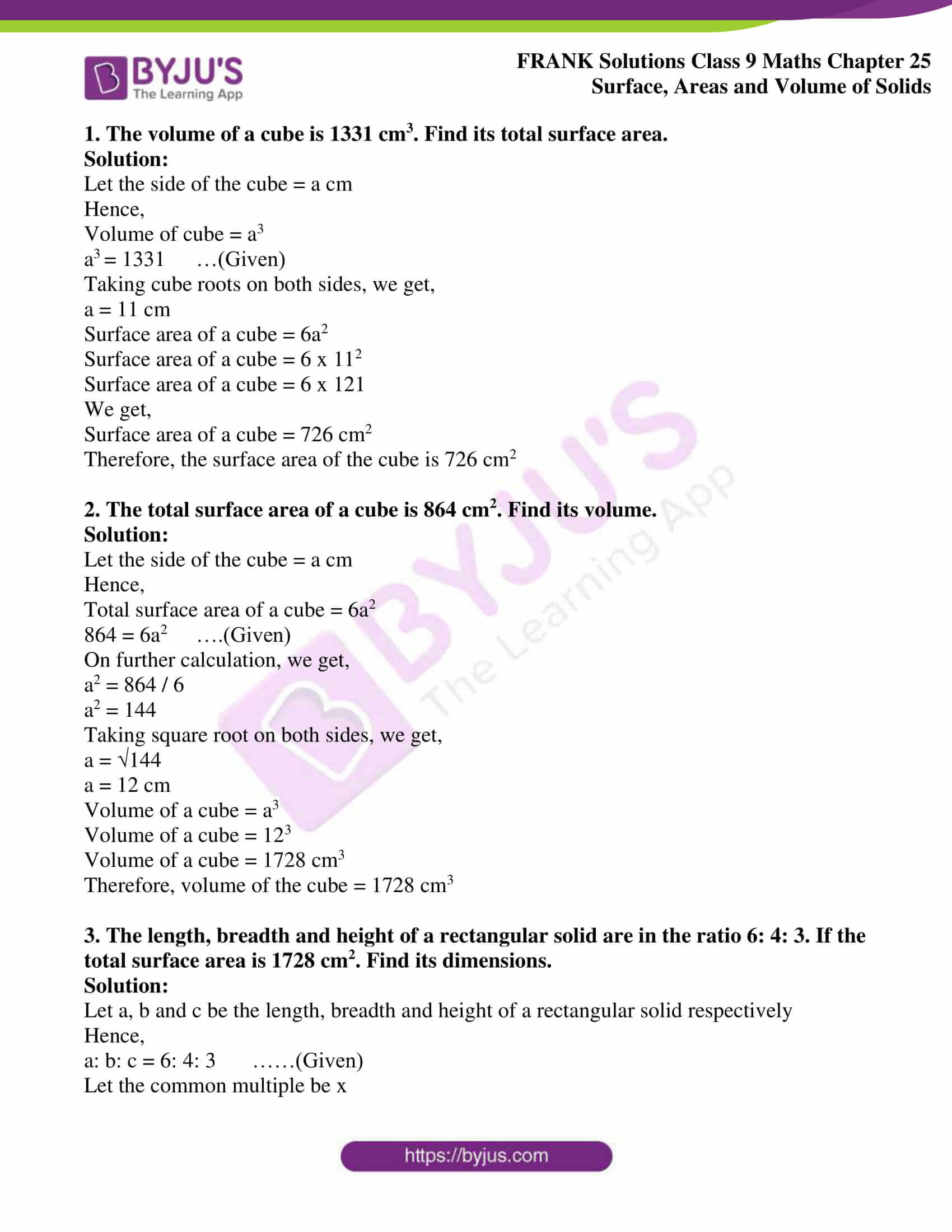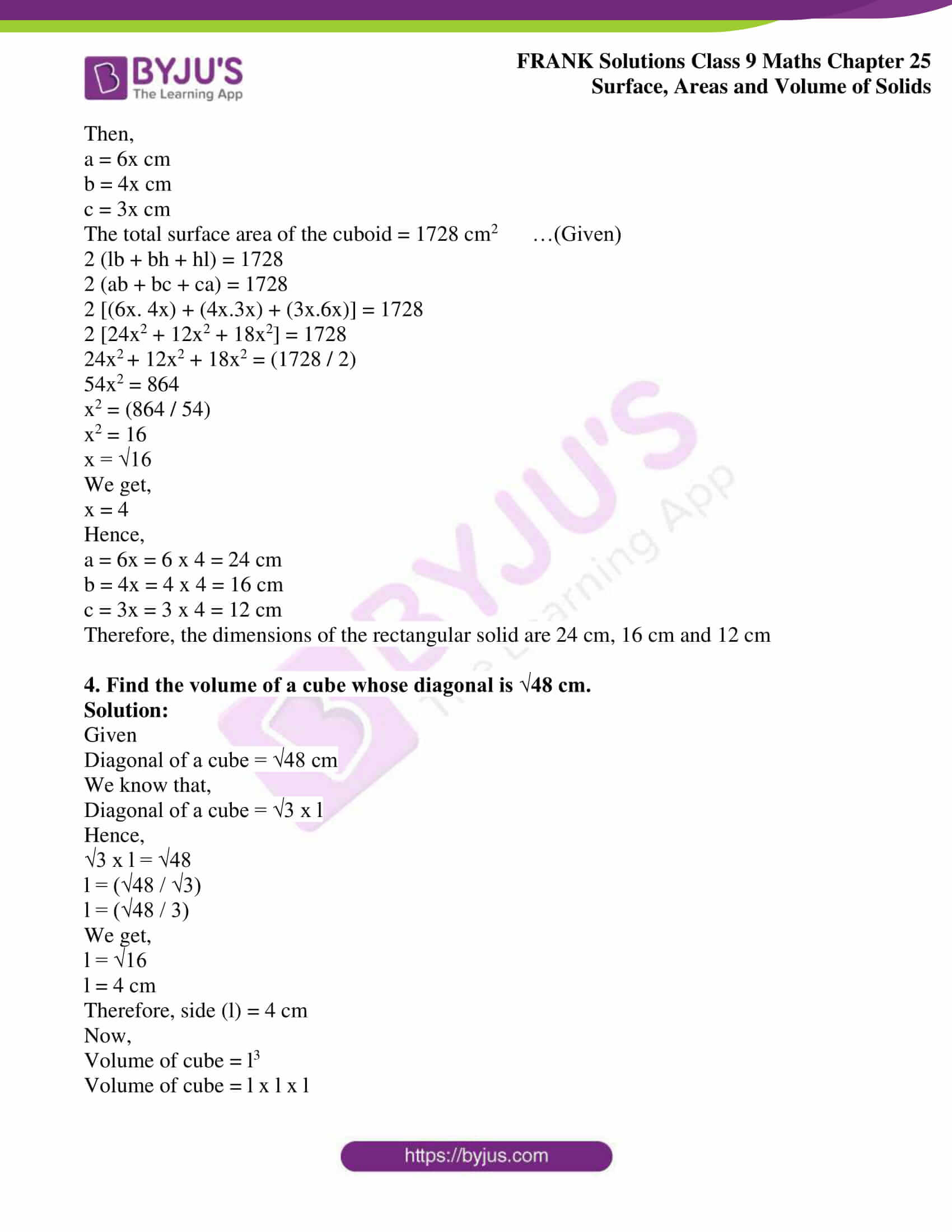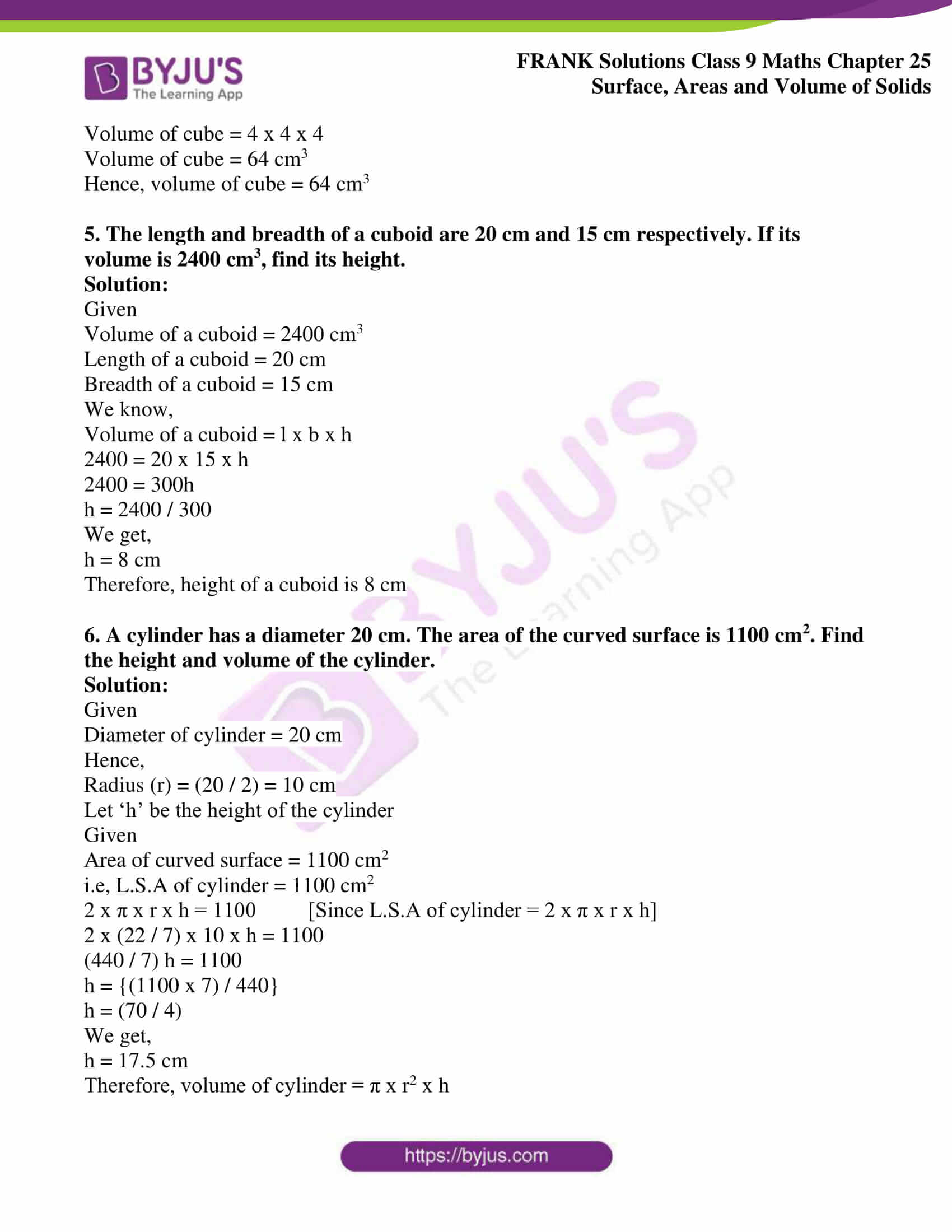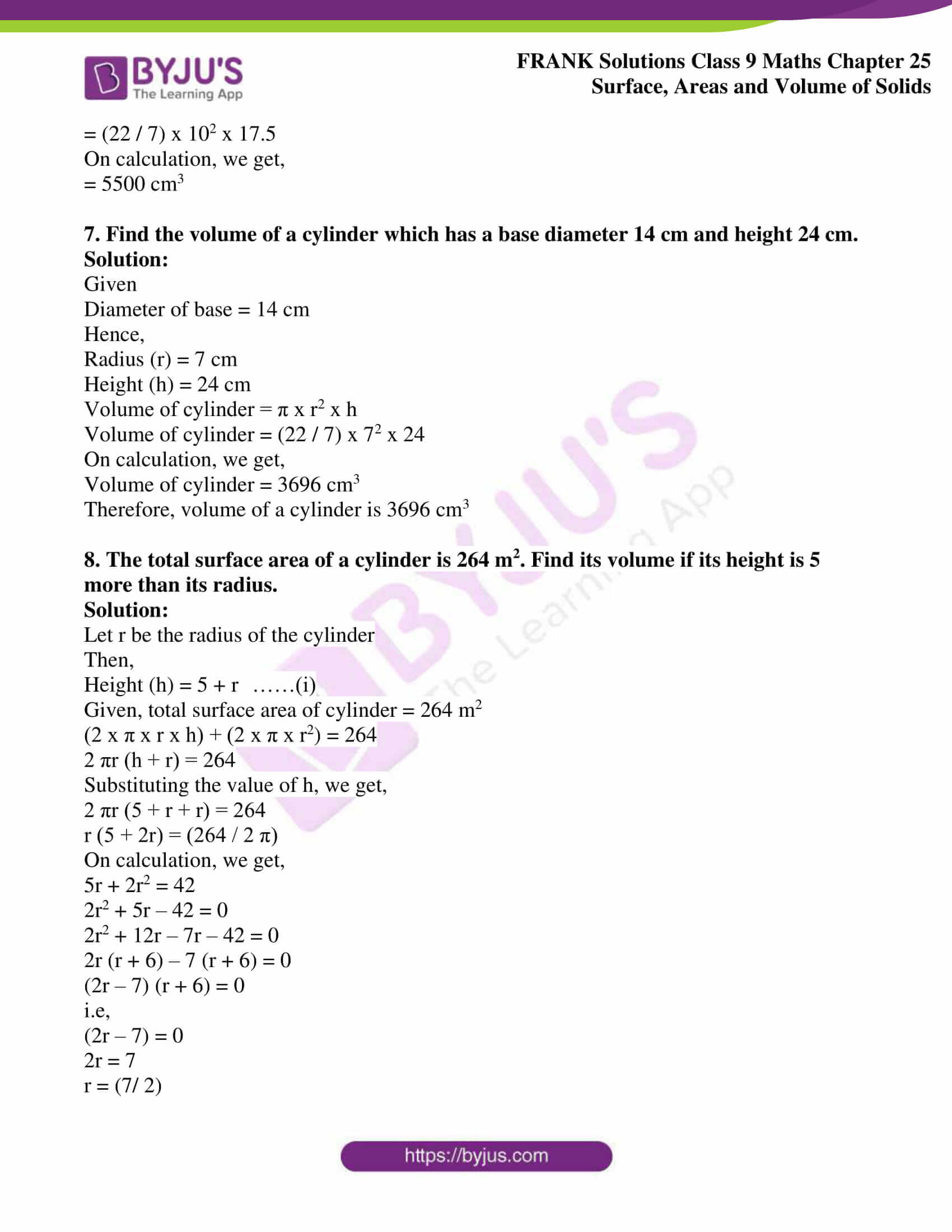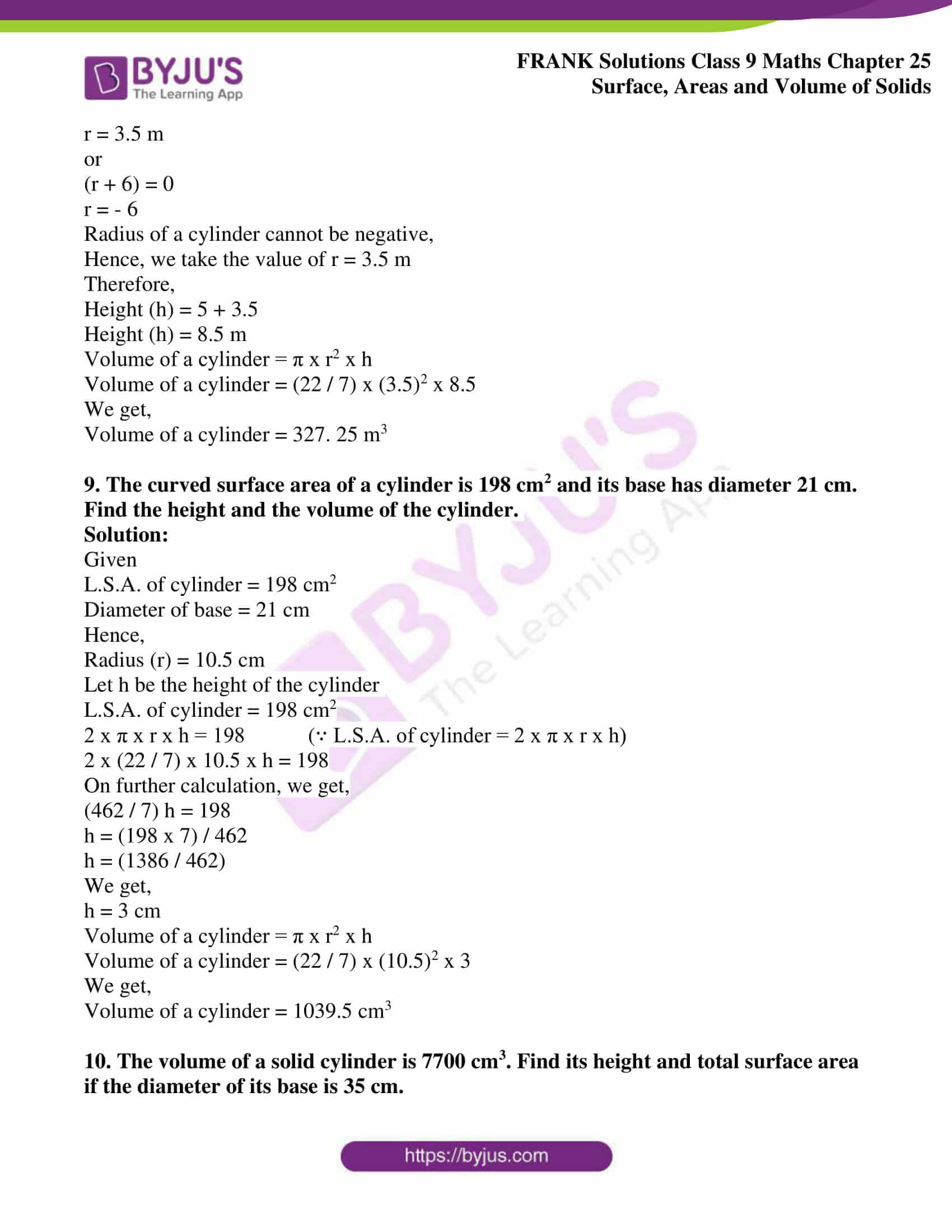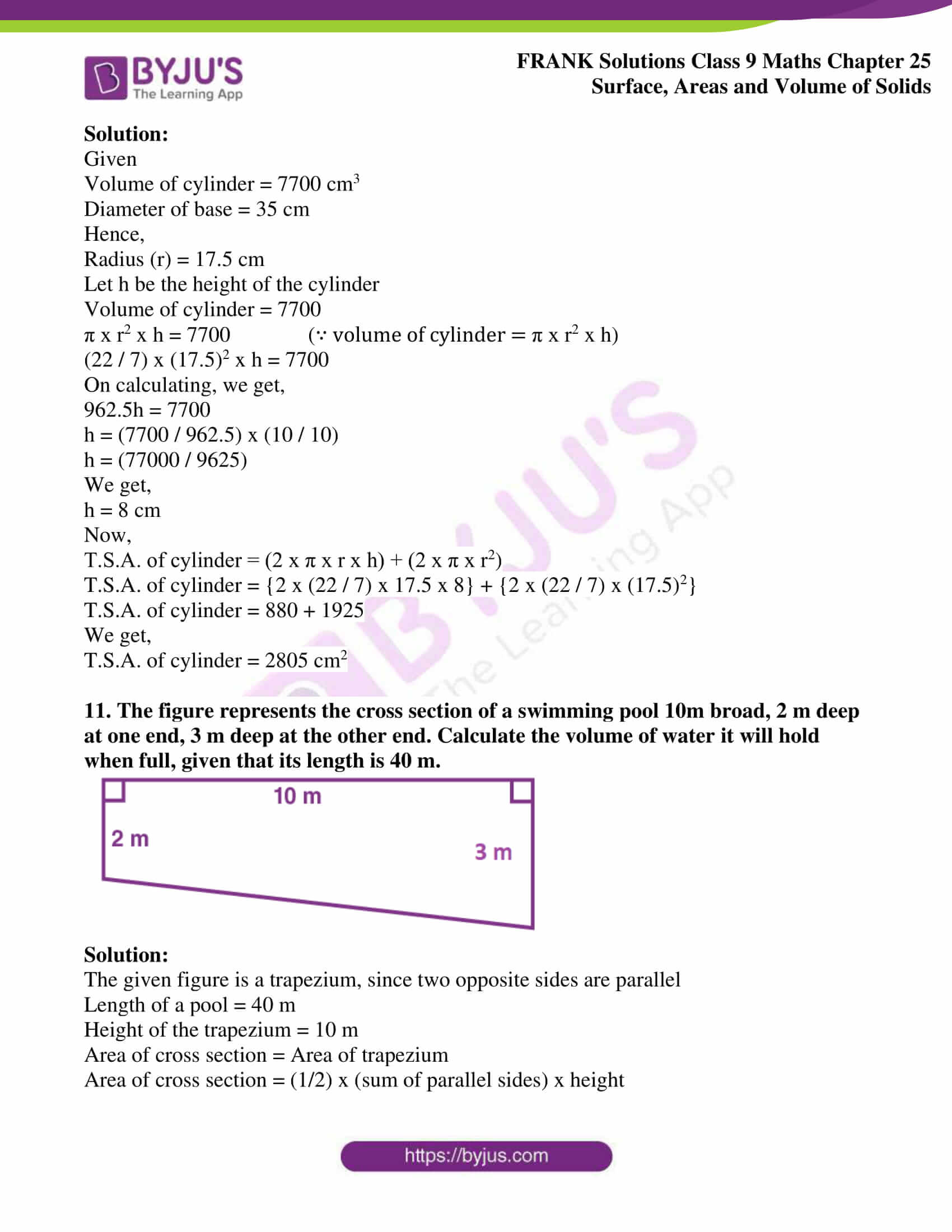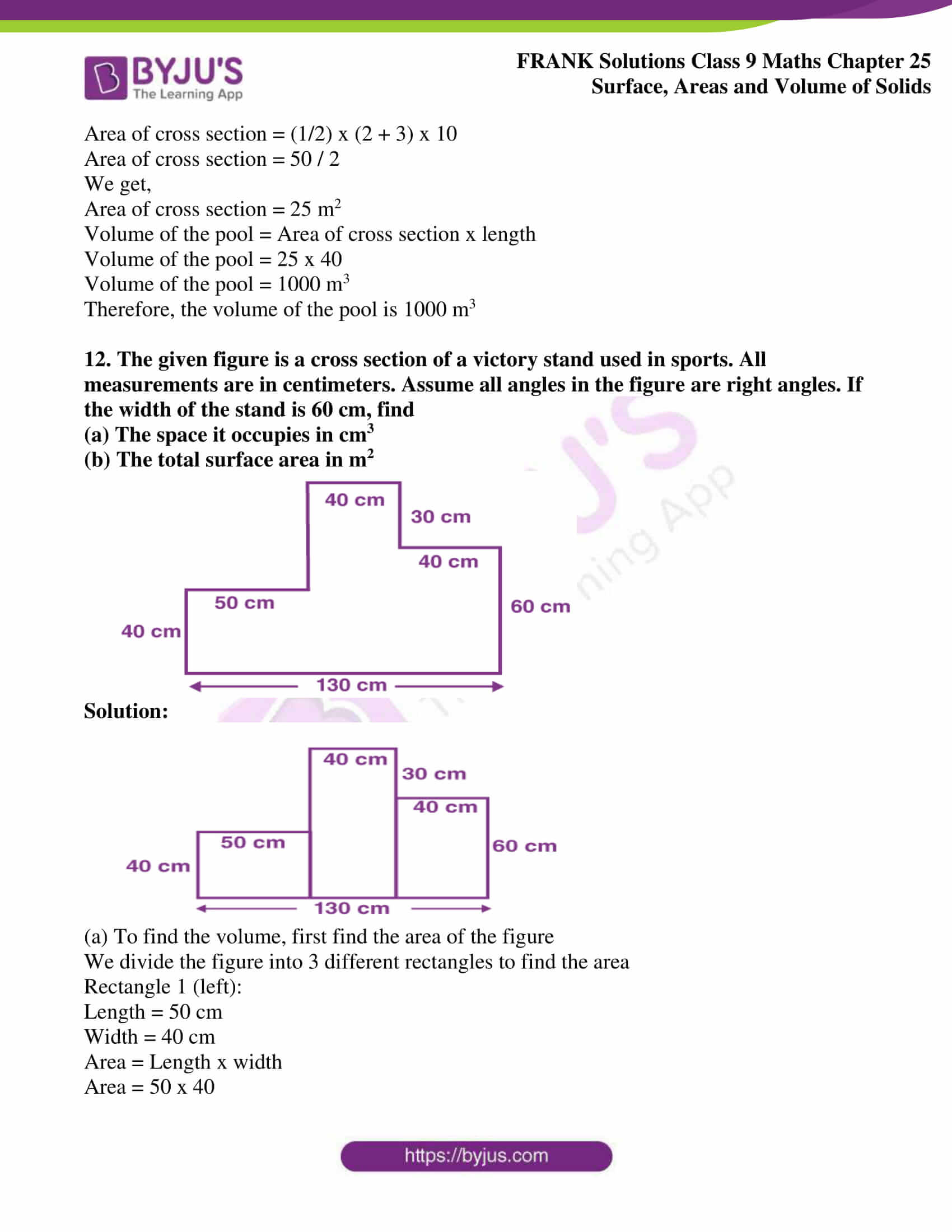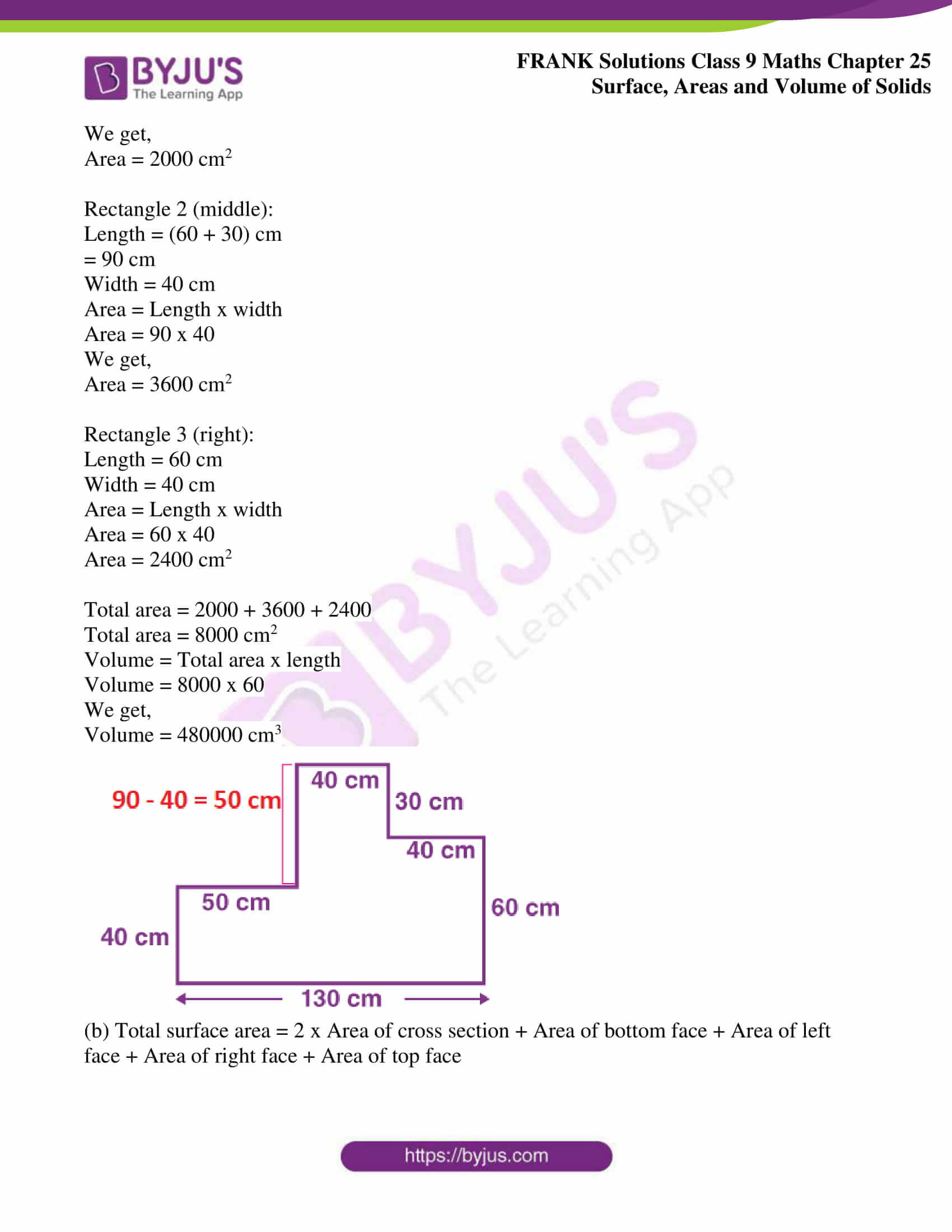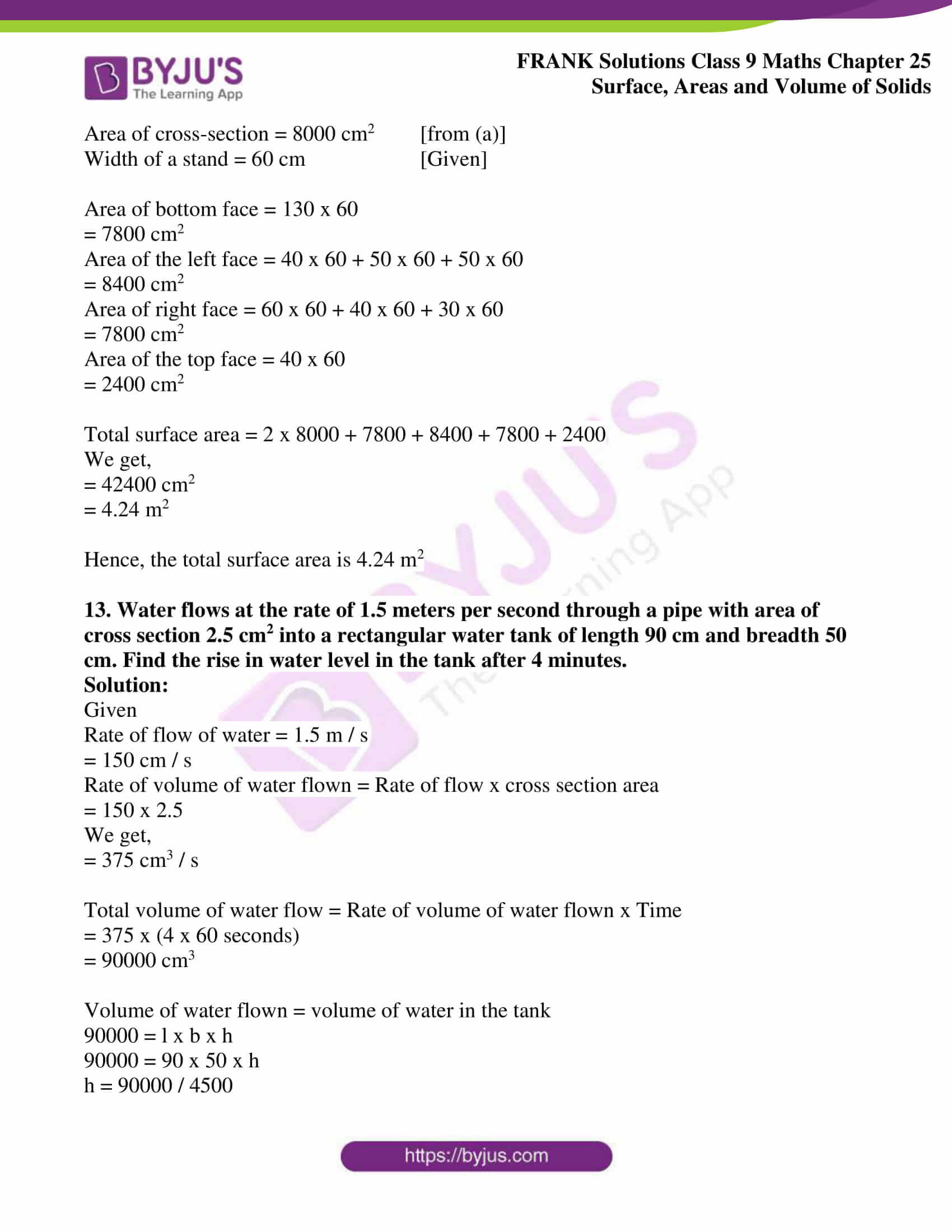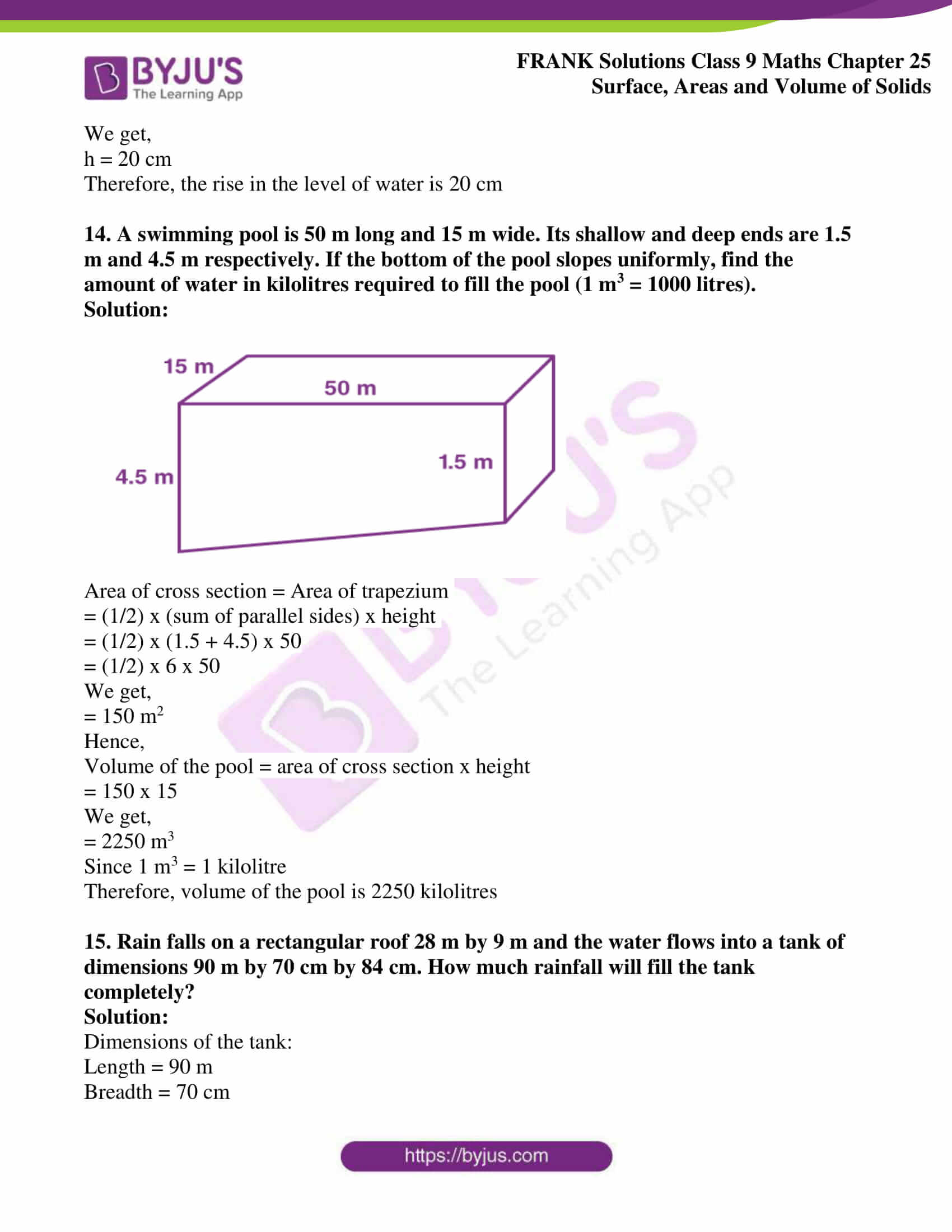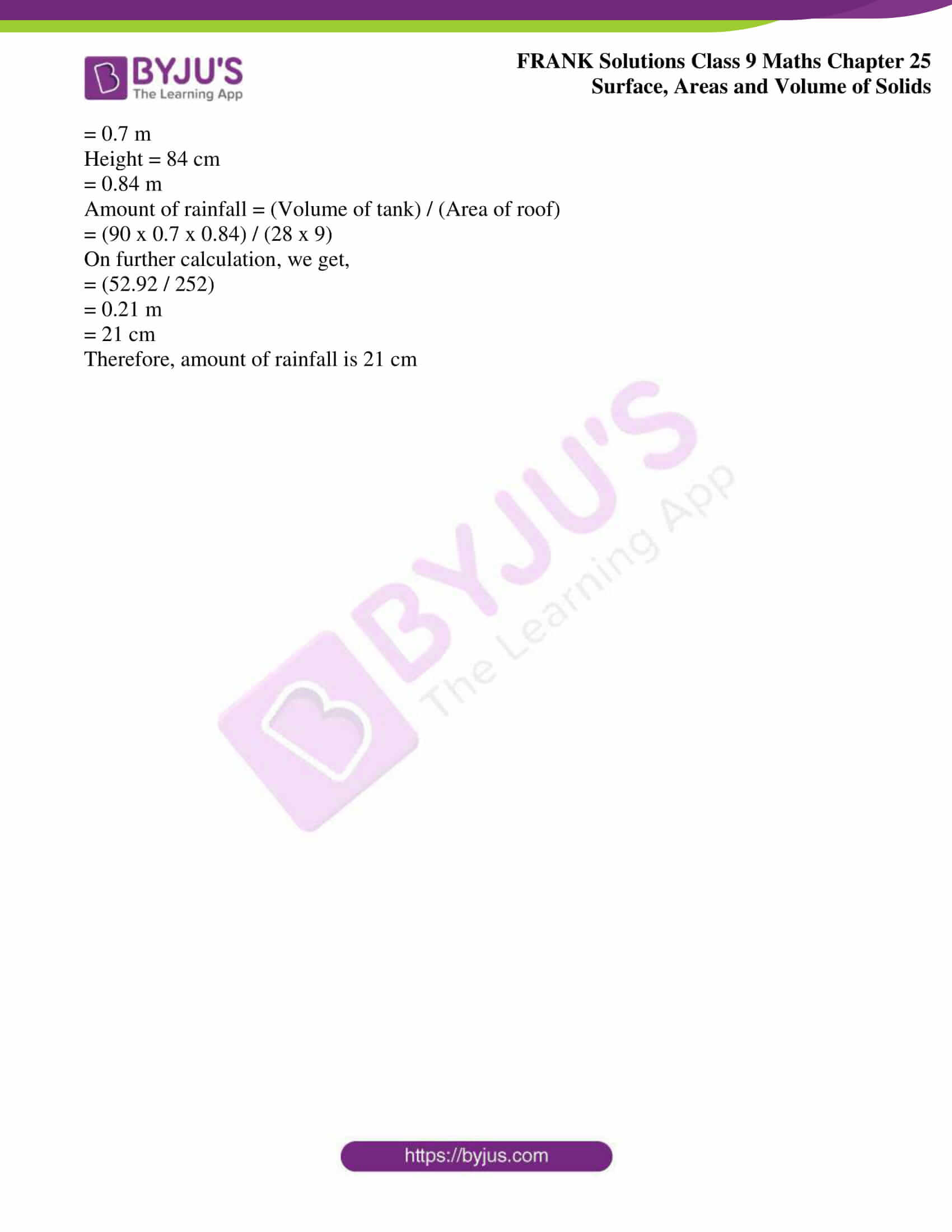## Access Frank Solutions for Class 9 Maths Chapter 25 Surface Areas and Volume of Solids

1. The volume of a cube is 1331 cm3. Find its total surface area.

Solution:

Let the side of the cube = a cm

Hence,

Volume of cube = a3

a3 = 1331 …(Given)

Taking cube roots on both sides, we get,

a = 11 cm

Surface area of a cube = 6a2

Surface area of a cube = 6 x 112

Surface area of a cube = 6 x 121

We get,

Surface area of a cube = 726 cm2

Therefore, the surface area of the cube is 726 cm2

2. The total surface area of a cube is 864 cm2. Find its volume.

Solution:

Let the side of the cube = a cm

Hence,

Total surface area of a cube = 6a2

864 = 6a2 ….(Given)

On further calculation, we get,

a2 = 864 / 6

a2 = 144

Taking square root on both sides, we get,

a = √144

a = 12 cm

Volume of a cube = a3

Volume of a cube = 123

Volume of a cube = 1728 cm3

Therefore, the volume of the cube = 1728 cm3

3. The length, breadth and height of a rectangular solid are in the ratio 6: 4: 3. If the total surface area is 1728 cm2. Find its dimensions.

Solution:

Let a, b and c be the length, breadth and height of a rectangular solid, respectively

Hence,

a: b: c = 6: 4: 3 ……(Given)

Let the common multiple be x

Then,

a = 6x cm

b = 4x cm

c = 3x cm

The total surface area of the cuboid = 1728 cm2 …(Given)

2 (lb + bh + hl) = 1728

2 (ab + bc + ca) = 1728

2 [(6x. 4x) + (4x.3x) + (3x.6x)] = 1728

2 [24x2 + 12x2 + 18x2] = 1728

24x2 + 12x2 + 18x2 = (1728 / 2)

54x2 = 864

x2 = (864 / 54)

x2 = 16

x = √16

We get,

x = 4

Hence,

a = 6x = 6 x 4 = 24 cm

b = 4x = 4 x 4 = 16 cm

c = 3x = 3 x 4 = 12 cm

Therefore, the dimensions of the rectangular solid are 24 cm, 16 cm and 12 cm

4. Find the volume of a cube whose diagonal is √48 cm.

Solution:

Given

Diagonal of a cube = √48 cm

We know that,

Diagonal of a cube = √3 x l

Hence,

√3 x l = √48

l = (√48 / √3)

l = (√48 / 3)

We get,

l = √16

l = 4 cm

Therefore, side (l) = 4 cm

Now,

Volume of cube = l3

Volume of cube = l x l x l

Volume of cube = 4 x 4 x 4

Volume of cube = 64 cm3

Hence, the volume of the cube = 64 cm3

5. The length and breadth of a cuboid are 20 cm and 15 cm, respectively. If its volume is 2400 cm3, find its height.

Solution:

Given

Volume of a cuboid = 2400 cm3

Length of a cuboid = 20 cm

Breadth of a cuboid = 15 cm

We know,

Volume of a cuboid = l x b x h

2400 = 20 x 15 x h

2400 = 300h

h = 2400 / 300

We get,

h = 8 cm

Therefore, height of a cuboid is 8 cm

6. A cylinder has a diameter 20 cm. The area of the curved surface is 1100 cm2. Find the height and volume of the cylinder.

Solution:

Given

Diameter of cylinder = 20 cm

Hence,

Radius (r) = (20 / 2) = 10 cm

Let ‘h’ be the height of the cylinder

Given

Area of curved surface = 1100 cm2

i.e, L.S.A of cylinder = 1100 cm2

2 x π x r x h = 1100 [Since L.S.A of cylinder = 2 x π x r x h]

2 x (22 / 7) x 10 x h = 1100

(440 / 7) h = 1100

h = {(1100 x 7) / 440}

h = (70 / 4)

We get,

h = 17.5 cm

Therefore, the volume of the cylinder = π x r2 x h

= (22 / 7) x 102 x 17.5

On calculation, we get,

= 5500 cm3

7. Find the volume of a cylinder which has a base diameter 14 cm and height 24 cm.

Solution:

Given

Diameter of base = 14 cm

Hence,

Height (h) = 24 cm

Volume of cylinder = π x r2 x h

Volume of cylinder = (22 / 7) x 72 x 24

On calculation, we get,

Volume of cylinder = 3696 cm3

Therefore, the volume of a cylinder is 3696 cm3

8. The total surface area of a cylinder is 264 m2. Find its volume if its height is 5 more than its radius.

Solution:

Let r be the radius of the cylinder

Then,

Height (h) = 5 + r ……(i)

Given, the total surface area of the cylinder = 264 m2

(2 x π x r x h) + (2 x π x r2) = 264

2 πr (h + r) = 264

Substituting the value of h, we get,

2 πr (5 + r + r) = 264

r (5 + 2r) = (264 / 2 π)

On calculation, we get,

5r + 2r2 = 42

2r2 + 5r – 42 = 0

2r2 + 12r – 7r – 42 = 0

2r (r + 6) – 7 (r + 6) = 0

(2r – 7) (r + 6) = 0

i.e,

(2r – 7) = 0

2r = 7

r = (7/ 2)

r = 3.5 m

or

(r + 6) = 0

r = – 6

Radius of a cylinder cannot be negative,

Hence, we take the value of r = 3.5 m

Therefore,

Height (h) = 5 + 3.5

Height (h) = 8.5 m

Volume of a cylinder = π x r2 x h

Volume of a cylinder = (22 / 7) x (3.5)2 x 8.5

We get,

Volume of a cylinder = 327. 25 m3

9. The curved surface area of a cylinder is 198 cm2, and its base has a diameter of 21 cm. Find the height and the volume of the cylinder.

Solution:

Given

L.S.A. of cylinder = 198 cm2

Diameter of base = 21 cm

Hence,

Let h be the height of the cylinder

L.S.A. of cylinder = 198 cm2

2 x π x r x h = 198 (∵ L.S.A. of cylinder = 2 x π x r x h)

2 x (22 / 7) x 10.5 x h = 198

On further calculation, we get,

(462 / 7) h = 198

h = (198 x 7) / 462

h = (1386 / 462)

We get,

h = 3 cm

Volume of a cylinder = π x r2 x h

Volume of a cylinder = (22 / 7) x (10.5)2 x 3

We get,

Volume of a cylinder = 1039.5 cm3

10. The volume of a solid cylinder is 7700 cm3. Find its height and total surface area if the diameter of its base is 35 cm.

Solution:

Given

Volume of cylinder = 7700 cm3

Diameter of base = 35 cm

Hence,

Let h be the height of the cylinder

Volume of cylinder = 7700

π x r2 x h = 7700 (∵ volume of cylinder = π x r2 x h)

(22 / 7) x (17.5)2 x h = 7700

On calculating, we get,

962.5h = 7700

h = (7700 / 962.5) x (10 / 10)

h = (77000 / 9625)

We get,

h = 8 cm

Now,

T.S.A. of cylinder = (2 x π x r x h) + (2 x π x r2)

T.S.A. of cylinder = {2 x (22 / 7) x 17.5 x 8} + {2 x (22 / 7) x (17.5)2}

T.S.A. of cylinder = 880 + 1925

We get,

T.S.A. of cylinder = 2805 cm2

11. The figure represents the cross-section of a swimming pool 10m broad, 2 m deep at one end, 3 m deep at the other end. Calculate the volume of water it will hold when full, given that its length is 40 m.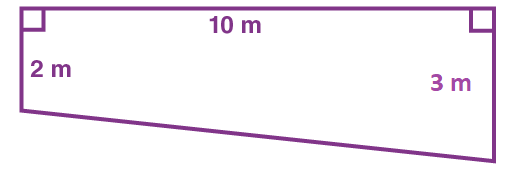Solution:

The given figure is a trapezium since two opposite sides are parallel

Length of a pool = 40 m

Height of the trapezium = 10 m

Area of cross-section = Area of trapezium

Area of cross-section = (1/2) x (sum of parallel sides) x height

Area of cross-section = (1/2) x (2 + 3) x 10

Area of cross-section = 50 / 2

We get,

Area of cross-section = 25 m2

Volume of the pool = Area of cross section x length

Volume of the pool = 25 x 40

Volume of the pool = 1000 m3

Therefore, the volume of the pool is 1000 m3

12. The given figure is a cross-section of a victory stand used in sports. All measurements are in centimetres. Assume all angles in the figure are right angles. If the width of the stand is 60 cm, find

(a) The space it occupies in cm3

(b) The total surface area in m2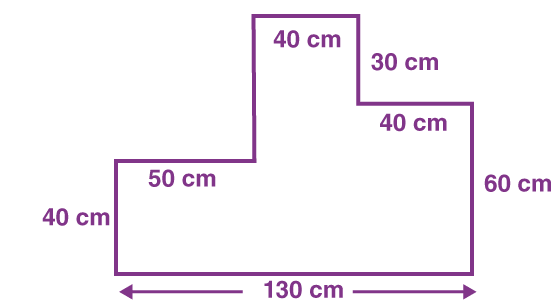Solution: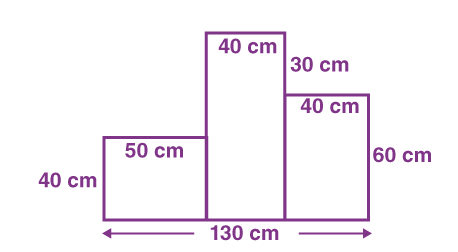(a) To find the volume, first, find the area of the figure

We divide the figure into 3 different rectangles to find the area

Rectangle 1 (left):

Length = 50 cm

Width = 40 cm

Area = Length x width

Area = 50 x 40

We get,

Area = 2000 cm2

Rectangle 2 (middle):

Length = (60 + 30) cm

= 90 cm

Width = 40 cm

Area = Length x width

Area = 90 x 40

We get,

Area = 3600 cm2

Rectangle 3 (right):

Length = 60 cm

Width = 40 cm

Area = Length x width

Area = 60 x 40

Area = 2400 cm2

Total area = 2000 + 3600 + 2400

Total area = 8000 cm2

Volume = Total area x length

Volume = 8000 x 60

We get,

Volume = 480000 cm3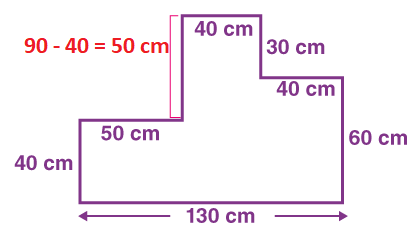(b) Total surface area = 2 x Area of cross section + Area of bottom face + Area of left face + Area of right face + Area of top face

Area of cross-section = 8000 cm2 [from (a)]

Width of a stand = 60 cm [Given]

Area of bottom face = 130 x 60

= 7800 cm2

Area of the left face = 40 x 60 + 50 x 60 + 50 x 60

= 8400 cm2

Area of right face = 60 x 60 + 40 x 60 + 30 x 60

= 7800 cm2

Area of the top face = 40 x 60

= 2400 cm2

Total surface area = 2 x 8000 + 7800 + 8400 + 7800 + 2400

We get,

= 42400 cm2

= 4.24 m2

Hence, the total surface area is 4.24 m2

13. Water flows at the rate of 1.5 meters per second through a pipe with an area of cross-section 2.5 cm2 into a rectangular water tank of length 90 cm and breadth 50 cm. Find the rise in water level in the tank after 4 minutes.

Solution:

Given

Rate of flow of water = 1.5 m / s

= 150 cm / s

Rate of volume of water flown = Rate of flow x cross-section area

= 150 x 2.5

We get,

= 375 cm3 / s

Total volume of water flow = Rate of volume of water flown x Time

= 375 x (4 x 60 seconds)

= 90000 cm3

Volume of water flown = volume of water in the tank

90000 = l x b x h

90000 = 90 x 50 x h

h = 90000 / 4500

We get,

h = 20 cm

Therefore, the rise in the level of water is 20 cm

14. A swimming pool is 50 m long and 15 m wide. Its shallow and deep ends are 1.5 m and 4.5 m, respectively. If the bottom of the pool slopes uniformly, find the amount of water in kilolitres required to fill the pool (1 m3 = 1000 litres).

Solution: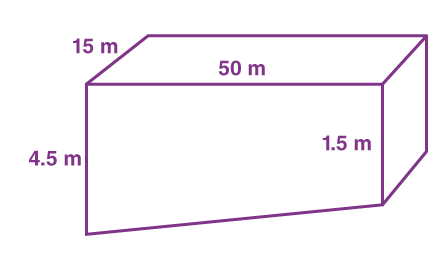Area of cross-section = Area of trapezium

= (1/2) x (sum of parallel sides) x height

= (1/2) x (1.5 + 4.5) x 50

= (1/2) x 6 x 50

We get,

= 150 m2

Hence,

Volume of the pool = area of cross section x height

= 150 x 15

We get,

= 2250 m3

Since 1 m3 = 1 kilolitre

Therefore, the volume of the pool is 2250 kilolitres.

15. Rain falls on a rectangular roof 28 m by 9 m, and the water flows into a tank of dimensions 90 m by 70 cm by 84 cm. How much rainfall will fill the tank completely?

Solution:

Dimensions of the tank:

Length = 90 m

= 0.7 m

Height = 84 cm

= 0.84 m

Amount of rainfall = (Volume of tank) / (Area of roof)

= (90 x 0.7 x 0.84) / (28 x 9)

On further calculation, we get,

= (52.92 / 252)

= 0.21 m

= 21 cm

Therefore, the amount of rainfall is 21 cm.